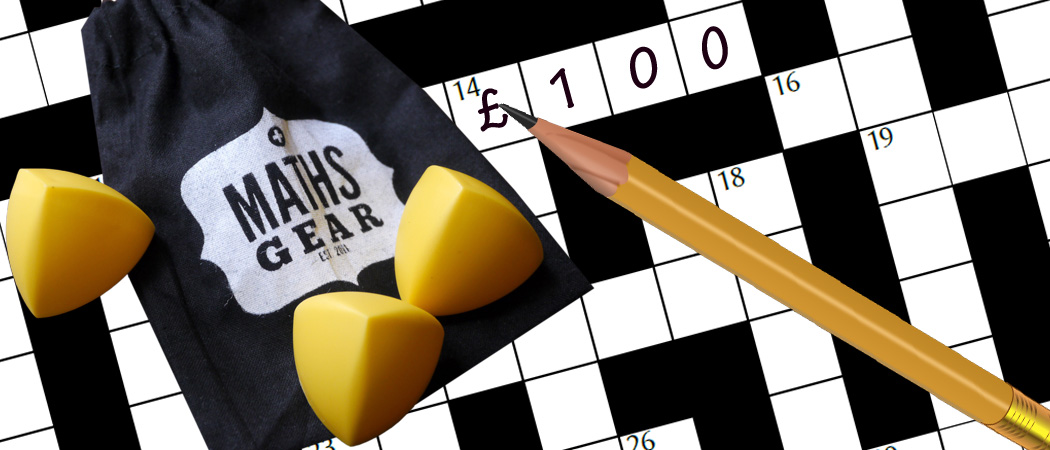# Prize crossnumber, Issue 14

Win a £100 Maths Gear goody bag by solving our infamous puzzleOur original prize crossnumber is featured on pages 60 and 61 of Issue 14.

### Rules

• The down clues are provided as normal. The across clues are provided in alphabetical order without their clue numbers or lengths.
• Although many of the clues have multiple answers, there is only one solution to the completed crossnumber. Solvers may wish to use the OEIS, Wikipedia, Python, a book of log tables, etc to (for example) obtain a list of cube numbers, but no programming should be necessary to solve the puzzle. As usual, no numbers begin with 0.
• One randomly selected correct answer will win a £100 Maths Gear goody bag, including non-transitive dice, a Festival of the Spoken Nerd DVD, and much, much more. Three randomly selected runners up will win a Chalkdust T-shirt. Maths Gear is a website that sells nerdy things worldwide, with free UK shipping.
• To enter, submit the sum of the across entries using this form by 14 March 2022. Only one entry per person will be accepted. Winners will be notified by email and announced on our blog by 1 May 2022.

### Crossnumber

Crossnumber #14, set by Humbug:### Across clues (see instructions)

• ? 217. (?)
• ? 394. (?)
• ? 555. (?)
• ? 777. (?)
• ? 790. (?)
• ? 935. (?)
• ? A factor of 2D. (?)
• ? A multiple of 51. (?)
• ? A power of 2. (?)
• ? A square number. (?)
• ? A square number. (?)
• ? An anagram of 25D. (?)
• ? Equal to 18D. (?)
• ? Equal to 34D. (?)
• ? Five less than 1A. (?)
• ? Four less than 1A. (?)
• ? One less than 1A. (?)
• ? One third of 6D. (?)
• ? Three less than 1A. (?)
• ? Two less than 1A. (?)
• ? Two times 4D. (?)

### Down clues

• 2. A square number. (2)
• 3. An anagram of 16D. (3)
• 4. Less than 38D. (2)
• 5. An even number. (3)
• 6. Two times 12D. (3)
• 7. Equal to 5D. (3)
• 9. A square number. (3)
• 11. A square number. (3)
• 12. Two times 18D. (3)
• 13. Equal to 5D. (3)
• 14. Less than 18D. (3)
• 15. A multiple of 23 whose digits are all odd. (3)
• 16. One less than 17A. (3)
• 18. Two times 14D. (3)
• 20. A palindromic prime number. (3)
• 22. A multiple of 23 whose digits are all odd. (3)
• 23. A multiple of 23 whose digits are all odd. (3)
• 24. Three times 30D. (3)
• 25. A multiple of 23 whose digits are all odd. (3)
• 27. 21A squared. (3)
• 29. A multiple of 25 whose digits are all odd. (3)
• 30. Three times 32D. (3)
• 31. One more than 3A. (3)
• 32. Less than 30D. (3)
• 33. A multiple of 23 whose digits are all odd. (3)
• 34. A cube number. (3)
• 36. 10 less than 2D. (2)
• 38. A multiple of 5. (2)